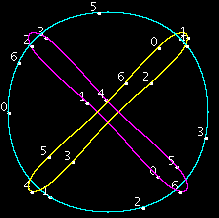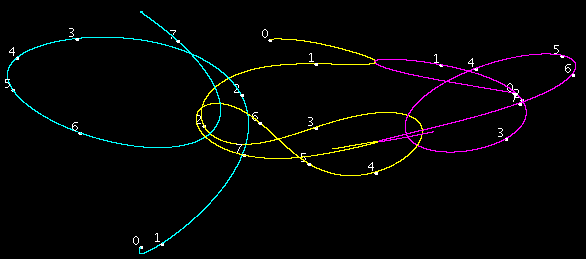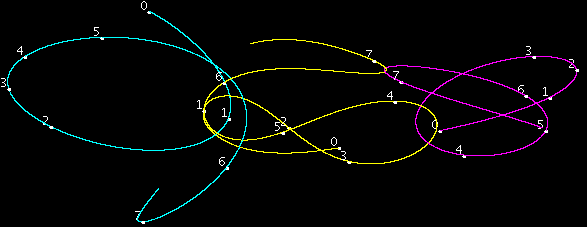### Runga-Kutta Orbit Calculations

This code computes the orbits of N particles in D dimensions in each other’s gravitaional field. D (number of dimensions) and N (number of point masses) are compile time parameters whose value is given in h.h.

The following commands compile and perform the 345 configuration to produce the images for the 345 problem:

```gcc p.c 345.c -std=c99 -O3 -lm
./a.out
javac r1.java
java Main
open *.png
rm trail* a.out Main.class```

m.c in place of 345.c tests each of the recent Periodic Three Body Solutions. This paper describes their discovery. I use routine solve in this package to gain more precision for these orbits.

Spin needs more parameters:

• File names for plotting.
I intend to keep D (number of dimensions) as a compile time parameter. I may make plotting a call-back.

This code computes and verifies circular, parabolic and elliptic orbits.
The Broucke-Hennon Orbits.
and perhaps the planets.

Just now, 2013 Apr 30, I (and others) have noticed that the 345 configuration leads to a period from t=8 thru 16 that is almost the time reversal of the period from t=16 thru 24. This may be adjustable to become a periodic palindromic solution where the particles all periodically and simultaneously come to rest.
8<=t<16 =>16<=t<24 =>